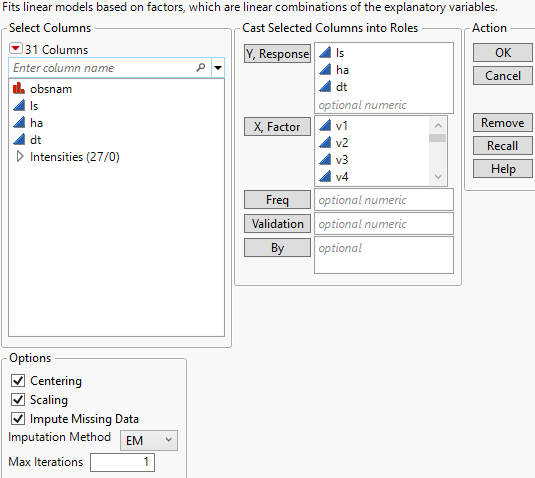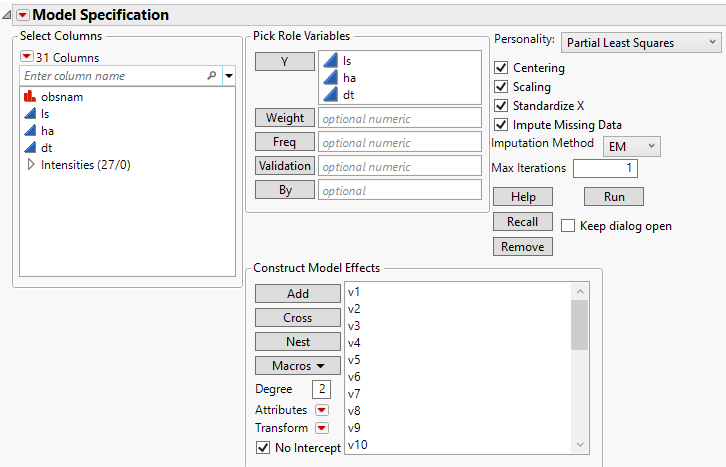Multivariate Methods > Partial Least Squares Models > Launch the Partial Least Squares Platform
Publication date: 11/10/2021

# Launch the Partial Least Squares Platform

There are two ways to launch the Partial Least Squares platform:

Select Analyze > Multivariate Methods > Partial Least Squares.Select Analyze > Fit Model and select Partial Least Squares from the Personality menu. This approach enables you to do the following:

Enter categorical variables as Ys or Xs. Conduct PLS-DA by entering categorical Ys.

Use the Standardize X option to construct higher-order terms using centered and scaled columns.

Some features on the Fit Model launch window are not applicable for the Partial Least Squares personality:

Weight, Nest, Attributes, Transform, and No Intercept.

Tip: You can transform a variable by right-clicking it in the Select Columns box and selecting a Transform option.

The following Macros: Mixture Response Surface, and Scheffé Cubic.

Figure 6.6 JMP Pro Partial Least Squares Launch Window (Imputation Method EM Selected)Figure 6.7 JMP Pro Fit Model Partial Least Squares Launch WindowFor more information about the Fit Model launch options, see Launch the Fit Model Platform in Fitting Linear Models.

The Partial Least Squares launch window contains the following options:

Y, Response

Enter numeric response columns. If you enter multiple columns, they are modeled jointly.In JMP Pro, you can enter nominal response columns in the Fit Model launch window to conduct PLS-DA. See PLS Discriminant Analysis (PLS-DA).

X, Factor

Enter the predictor columns. The Partial Least Squares launch window allows only numeric predictors.In JMP Pro, you can enter nominal and ordinal model effects in the Fit Model launch window. Ordinal effects are treated as nominal.

Freq

If your data are summarized, enter the column whose values contain counts for each row.Validation

A numeric column that defines the validation sets. A validation column must contain only consecutive integer values:

If the validation column has two levels, the smaller value defines the training set and the larger value defines the validation set.

If the validation column has three levels, the values define the training, validation, and test sets in order of increasing size.

If the validation column has more than three levels, then KFold Cross Validation is used. For information about other validation options, see Validation Method.

The PLS platform uses the validation column to train and tune the model or to train, tune, and evaluate the model. For more information about validation, see Validation in JMP Modeling.

Note: If you click the Validation button with no columns selected in the Select Columns list, you can add a validation column to your data table. For more information about the Make Validation Column utility, Make Validation Column in Predictive and Specialized Modeling.

By

Enter a column that creates a report consisting of separate analyses for each level of the variable. If more than one By variable is assigned, a separate analysis is produced for each possible combination of the levels of the By variables.

Centering

Centers all Y variables and model effects by subtracting the mean from each column. See Centering and Scaling.

Scaling

Scales all Y variables and model effects by dividing each column by its standard deviation. See Centering and Scaling.Standardize X

(Available only in the Fit Model launch window.) Centers and scales all columns that are used in the construction of model effects. If this option is not selected, higher-order effects are constructed using the original data table columns. Then each higher-order effect is centered or scaled, based on the selected Centering and Scaling options. Note that Standardize X does not center or scale Y variables. See Standardize X.Impute Missing Data

Replaces missing data values in Ys or Xs with nonmissing values. Select the appropriate method from the Imputation Method list.

If Impute Missing Data is not selected, rows that are missing observations on any X variable are excluded from the analysis and no predictions are computed for these rows. Rows with no missing observations on X variables but with missing observations on Y variables are also excluded from the analysis, but predictions are computed.Imputation Method

(Appears only when Impute Missing Data is selected.) Select from the following imputation methods:

Mean

For each model effect or response column, replaces the missing value with the mean of the nonmissing values.

EM

Uses an iterative Expectation-Maximization (EM) approach to impute missing values. On the first iteration, the specified model is fit to the data with missing values for an effect or response replaced by their means. Predicted values from the model for Y and the model for X are used to impute the missing values. For subsequent iterations, the missing values are replaced by their predicted values, given the conditional distribution using the current estimates.

For the purpose of imputation, polynomial terms are treated as separate predictors. When a polynomial term is specified, that term is calculated from the original data, or, if Standardize X is checked, from the standardized column values. If a row has a missing value for a column involved in the definition of the polynomial term, then that entry is missing for the polynomial term. Imputation is conducted using polynomial terms defined in this way.Max Iterations